# Place Value, Rounding, Addition, and Subtraction

Students build on their understanding of the structure of the place value system, start to use rounding as a way to estimate quantities, and develop fluency with the standard algorithm of addition and subtraction. Students focus on the precision of their calculations, and use them to solve real-world problems.

## Unit Summary

In the first unit of Grade 3, students will build on their understanding of the structure of the place value system from Grade 2 (MP.7), start to use rounding as a way to estimate quantities (3.NBT.1), as well as develop fluency with the standard algorithm of addition and subtraction (3.NBT.2). Throughout the unit, students attend to the precision of their calculations (MP.6) and use them to solve real-world problems (MP.4).

In Grade 2, students developed an understanding of the structure of the base-ten system as based in repeated bundling in groups of 10. With this deepened understanding of the place value system, Grade 2 students “add and subtract within 1000, with composing and decomposing, and they understand and explain the reasoning of the processes they use” (NBT Progressions, p. 8). These processes and strategies include concrete models or drawings and strategies based on place value, properties of operations, and/or the relationship between addition and subtraction (2.NBT.7). As such, at the end of Grade 2, students are able to add and subtract within 1,000 but often aren’t relying on the standard algorithm to solve.

Thus, Unit 1 starts off with reinforcing some of this place value understanding of thousands, hundreds, tens, and ones being made up of 10 of the unit to its right that students learned in Grade 2. Students use this sense of magnitude and the idea of benchmark numbers to first place numbers on number lines of various endpoints and intervals, and next use those number lines as a model to help students round two-digit numbers to the tens place as well as three-digit numbers to the hundreds and tens place (3.NBT.1). Next, students focus on developing their fluency with the addition and subtraction algorithms up to 1,000, making connections to the place value understandings and other models they learned in Grade 2 (3.NBT.2). Last, the unit culminates in a synthesis of all learning thus far in the unit, in which students solve one- and two-step word problems involving addition and subtraction and use rounding to assess the reasonableness of their answer (3.OA.8), connecting the NBT and OA domains. These skills are developed further and built upon in subsequent units in which multiplication and division are added to the types of word problems students estimate and solve.

This builds toward an even deeper understanding of the place value system that students learn in Grade 4. In Grade 4, students learn about multiplicative comparison; i.e.: a value being x times as many as another value. Thus, students’ understanding of the place value system is more precisely refined as “a digit in one place represents ten times what it represents in the place to its right” (4.NBT.1, emphasis ours). Further, students learn to round any multi-digit number to any place. They also use the standard algorithm to solve addition and subtraction problems to the new place values they encounter at this grade level, namely, to one million. Thus, while the majority of the content learned in this unit comes from an additional cluster, they are deeply important skills necessary to fully master the major work of the grade with 3.OA.8, as well as a foundation for rounding and the standard algorithms used to any place value learned in Grade 4 (4.NBT.1—4) and depended on for many grade levels after that.

Pacing: 16 instructional days (14 lessons, 1 flex day, 1 assessment day)

For guidance on adjusting the pacing for the 2021-2022 school year, see our 3rd Grade Scope and Sequence Recommended Adjustments.• Expanded Assessment Package
• Problem Sets for Each Lesson
• Student Handout Editor
• Vocabulary Package

## Assessment

This assessment accompanies Unit 1 and should be given on the suggested assessment day or after completing the unit.

## Unit Prep

### Intellectual Prep

?

#### Intellectual Prep for All Units

• Read and annotate “Unit Summary” and “Essential Understandings” portion of the unit plan.
• Do all the Target Tasks and annotate them with the “Unit Summary” and “Essential Understandings” in mind.
• Take the Post-Unit Assessment.

#### Unit-Specific Intellectual Prep

• Read the following table that includes models used in this unit.
 Pictorial or concrete base ten blocks Example: Represent 342 with base ten blocks.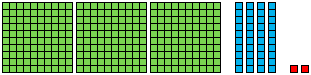Number line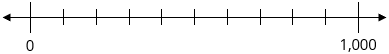Standard algorithm for addition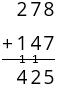Standard algorithm for subtraction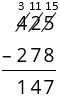Tape diagram Example: A grocery store sells 172 red apples and 86 green apples. How many apples did the grocery store sell?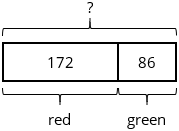### Essential Understandings

?

• The rounding process is based on knowing the number halfway between multiples of 10, 100, and so on. Rounding is a process for finding the multiple of 10, 100, etc. closest to a given number.
• Fluently adding and subtracting depends on a deep knowledge of the place value system and flexibility in solving. The numbers themselves should dictate which strategy is used, as sometimes that may be the standard algorithm, and other times it may be a mental strategy (such as converting 199 + 456 to 200 + 455).
• Rounding values before computing with them can result in an estimate that is either too high or too low, depending on how the numbers were originally rounded, what computation is being performed, and where in the number sentence it’s located.
• Making sense of problems and persevering to solve them is an important practice when solving word problems. Key words do not always indicate the correct operation.

### Materials

?

• Tic-Tac-Toe Boards (1 per pair of students)
• Markers or crayons (2 of different colors per pair of students) — Students could use a pen and a pencil instead.
• Optional: Paper base ten blocks (Total of 9 per student or small group) — Students might not need these depending on their reliance on concrete materials.
• Optional: Base ten blocks (Maximum of about 5 hundreds, 20 tens, 20 ones per student or small group) — Students might not need these depending on their reliance on concrete materials. Or, students can use Paper base ten blocks cut into units instead.
• Thousands Place Value Chart (Total of 6 per student) — Students might need more or less depending on their reliance on this tool.

### Vocabulary

?

whole

${\approx}$

endpoint

algorithm

value

round

reasonable

place

number line

interval

estimate/estimation

digit

approximate/approximation

To see all the vocabulary for this course, view our 3rd Grade Vocabulary Glossary.#### Unit Practice

With Fishtank Plus you can access our Daily Word Problem Practice and our content-aligned Fluency Activities created to help students strengthen their application and fluency skills.

View Preview

# 1

3.NBT.A.1

3.NBT.A.2

Use counting, place value understanding, and addition/subtraction of ones and tens to complete a partially filled-in number grid.

# 2

3.NBT.A.1

3.NBT.A.2

Represent three-digit numbers using concrete manipulatives and drawings, including cases with more than 9 of any unit.

# 3

3.NBT.A.1

Locate three-digit numbers on a number line and explain their placement.

# 4

3.NBT.A.1

Define estimation and its purpose. Round two-digit numbers to the nearest ten using a number line.

# 5

3.NBT.A.1

Round three-digit numbers to the nearest hundred using a number line.

# 6

3.NBT.A.1

Round three-digit numbers to the nearest ten using a number line.

# 7

3.NBT.A.1

Round multi-digit numbers to any place in more complex cases, including those involving real-world contexts and/or assessing the reasonableness of that estimate.

# 8

3.NBT.A.2

Add two numbers with up to one composition within 1,000.

# 9

3.NBT.A.2

Add two numbers with multiple compositions within 1,000.

# 10

3.NBT.A.1

3.NBT.A.2

3.OA.D.8

Solve word problems involving addition, using rounding to assess the reasonableness of answers.

# 11

3.NBT.A.2

Subtract two numbers with up to one decomposition within 1,000.

# 12

3.NBT.A.2

Subtract two numbers with multiple decompositions within 1,000.

# 13

3.NBT.A.1

3.NBT.A.2

3.OA.D.8

Solve word problems involving subtraction, using rounding to assess the reasonableness of answers.

# 14

3.NBT.A.1

3.NBT.A.2

3.OA.D.8

Solve one- and two-step word problems involving addition and subtraction, using rounding to assess the reasonableness of answers.

## Common Core Standards

Key: Major Cluster Supporting Cluster Additional Cluster

### Core Standards

?

##### Number and Operations in Base Ten
• 3.NBT.A.1 — Use place value understanding to round whole numbers to the nearest 10 or 100.

• 3.NBT.A.2 — Fluently add and subtract within 1000 using strategies and algorithms based on place value, properties of operations, and/or the relationship between addition and subtraction.

##### Operations and Algebraic Thinking
• 3.OA.D.8 — Solve two-step word problems using the four operations. Represent these problems using equations with a letter standing for the unknown quantity. Assess the reasonableness of answers using mental computation and estimation strategies including rounding. This standard is limited to problems posed with whole numbers and having whole-number answers; students should know how to perform operations in the conventional order when there are no parentheses to specify a particular order (Order of Operations).

?

• 2.MD.B.6

• 2.NBT.A.1

• 2.NBT.A.2

• 2.NBT.A.3

• 2.NBT.B.5

• 2.NBT.B.7

• 2.NBT.B.8

• 2.NBT.B.9

• 2.OA.A.1

?

• 4.NBT.A.3

• 4.NBT.B.4

### Standards for Mathematical Practice

• CCSS.MATH.PRACTICE.MP1 — Make sense of problems and persevere in solving them.

• CCSS.MATH.PRACTICE.MP2 — Reason abstractly and quantitatively.

• CCSS.MATH.PRACTICE.MP3 — Construct viable arguments and critique the reasoning of others.

• CCSS.MATH.PRACTICE.MP4 — Model with mathematics.

• CCSS.MATH.PRACTICE.MP5 — Use appropriate tools strategically.

• CCSS.MATH.PRACTICE.MP6 — Attend to precision.

• CCSS.MATH.PRACTICE.MP7 — Look for and make use of structure.

• CCSS.MATH.PRACTICE.MP8 — Look for and express regularity in repeated reasoning.